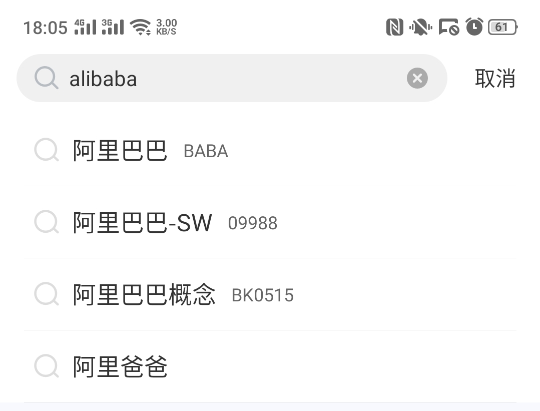# 干货|app自动化之如何参数化用例

## 使用方法

``````@pytest.mark.parametrize("argvnames",argvalues)

``````

@pytest.mark.parametrize() 需要传入两个参数 “argnamest” 与 “argvalues”，第一个参数需要一个或者多个变量来接收列表中的每组数据，第二个参数传递存储数据的列表。测试用例需要使用同名的字符串接收测试数据（与“argvnames”里面的名字一致），且列表有多少个元素就会生成并执行多个测试用例。下面示例使用使用参数化定义三组数据，每组数据都存放在一个元组中，分别将元组中的数据传入（test_input,expected）参数中，示例代码如下：

``````# content of test_expectation.py
import pytest

@pytest.mark.parametrize("test_input,expected", [("3+5", 8), ("2+4", 6), ("6*9", 42)])
def test_eval(test_input, expected):
assert eval(test_input) == expected

``````

``````...
test_expectation.py ..F

test_input = '6*9', expected = 42

@pytest.mark.parametrize("test_input,expected",
[("3+5", 8), ("2+4", 6), ("6*9", 42)])

def test_eval(test_input, expected):
>       assert eval(test_input) == expected
E       AssertionError: assert 54 == 42
E        +  where 54 = eval('6*9')

test_expectation.py:6: AssertionError

``````

## 案例``````from appium import webdriver
import pytest
from hamcrest import *

class TestXueqiu:
省略...
# 参数化
@pytest.mark.parametrize("keyword, stock_type, expect_price", [
('alibaba', 'BABA', 170),
('xiaomi', '01810', 8.5)
])
def test_search(self, keyword, stock_type, expect_price):
# 点击搜索
self.driver.find_element_by_id("home_search").click()
# 向搜索框中输入keyword
self.driver.find_element_by_id(
"com.xueqiu.android:id/search_input_text"
).send_keys(keyword)

# 点击搜索结果
self.driver.find_element_by_id("name").click()
# 获取价格
price = float(self.driver.find_element_by_xpath(
"//*[contains(@resource-id, 'stockCode')\
and @text='%s']/../../..\
//*[contains(@resource-id, 'current_price')]"
% stock_type
).text)
# 断言
assert_that(price, close_to(expect_price, expect_price * 0.1))

``````

app自动化测试（Android）参数化用例就讲完了，大家学会了么？我们下一期为大家讲解app自动化中Andriod WebView测试，有兴趣的小伙伴可以关注一下哦！